Next: Model Equivalence Up: Models Previous: Single-level GLM

## Estimation

The BLUEs for both the two-level GLM and the single-level GLM can be calculated using the General Least Squares approach .

Initially consider the two-level GLM. The parameter estimates at the first level are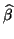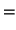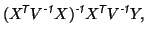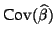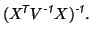(5)

Similarly, the estimates of the (second-level) group parameters are given by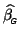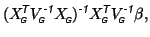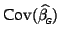In practice, however, the second-level model uses the estimates from the first level as input and not the true (but unobservable) parameters. That is, equation 2 is modified, becoming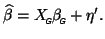(6)

Therefore the two-level model, as used in practice, is specified by equations 1 and 6. This has significant implications, as the two-level version is no longer precisely equivalent to the single-level model in terms of estimation. In particular, the estimation of the group parameters in the two-level model now is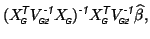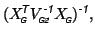(7)

where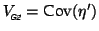represents the potentially different covariance in this new two-level model.

Now consider the single-level GLM (equation 4), where the BLUE is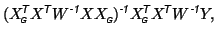(8)

This equation directly relates the group parameter estimates of interest,, to the full data vector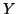and so requires the GLM to be solved for matrices of greatly increased size.

Thus, instead of solving the single-level model all at once, we wish to use the two-level approach. However, substituting equation 5 into equation 7 gives the two-level group parameter estimates as: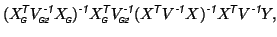(9)

If this estimation (equation 9) can be made equivalent to the single-level estimation (equation 8) by accounting for the covariances of the first-level estimates within the second-level (i.e. setting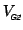appropriately), then the two approaches become exactly equivalent. This turns out to be possible and the general equivalence result is presented in the next section.Next: Model Equivalence Up: Models Previous: Single-level GLM
Christian Beckmann 2003-07-16